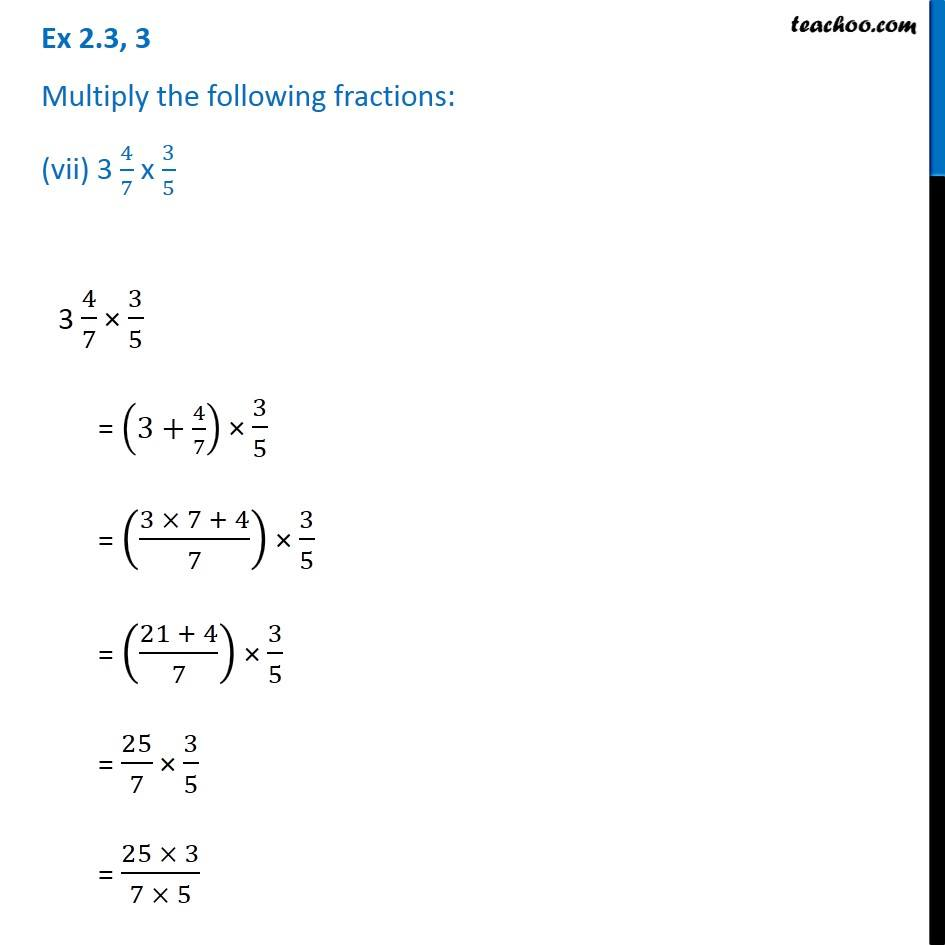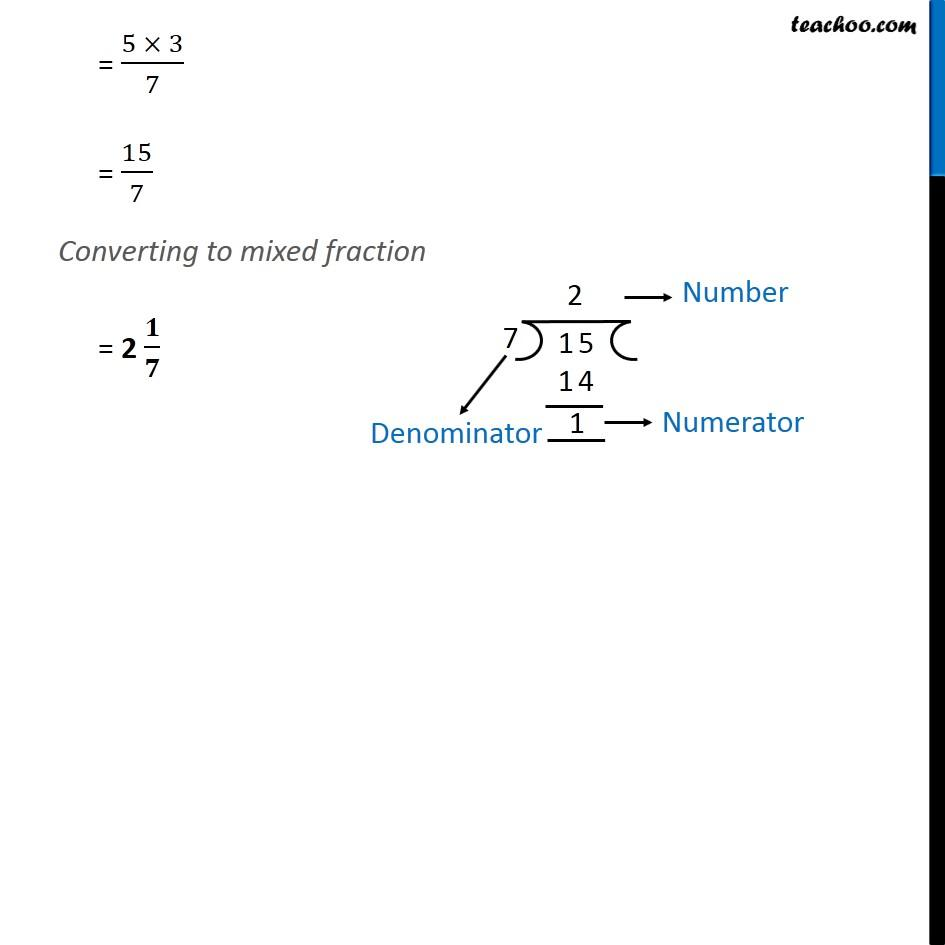1. Chapter 2 Class 7 Fractions and Decimals
2. Serial order wise
3. Ex 2.3

Transcript

Ex 2.3, 3 Multiply the following fractions: (vii) 3 4/7 x 3/5 3 4/7 × 3/5 = (3+4/7) × 3/5 = ((3 × 7 + 4)/7) × 3/5 = ((21 + 4)/7) × 3/5 = 25/7 × 3/5 = (25 × 3)/(7 × 5) = (5 × 3)/7 = 15/7 Converting to mixed fraction = 2 𝟏/𝟕